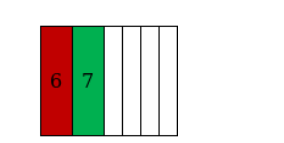# How many 6-digit telephone numbers can be constructed using the digits

Question:

How many 6-digit telephone numbers can be constructed using the digits 0 to 9, if each number starts with 67 and no digit appears more than once?

Solution:

To find: 6-digit telephone numbers that can be constructed using the digits 0 to 9.

Condition: each number starts with 67 and no digit appears more than onceThere are 10 digits between 0 to 9 , and two of them are utilized in filling up the first two digits i.e. 67 of the 6 digit phone number, so remaining number of digits $=10-2=8$, and this need to be used in filling up the remaining $6-2=4$ places of the telephone number.

So, number of ways $=8 \times 7 \times 6 \times 5=1680$.